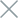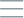• Home
• Tags
• Series
•# Testing Functions and Methods with Chai

## Testing functions for throwing the correct exceptions

To test for exceptions, we can use the `throw` assertion provided by Chai. This assertion allows us to specify the type of exception that should be thrown and any additional details we want to validate. By including this assertion in our test cases, we can ensure that our functions behave as expected and handle error conditions appropriately.

Let's consider an example where we have a function that divides two numbers. We want to ensure that the function throws an exception when dividing by zero. We can write a test case using Chai's `throw` assertion to check if the function correctly throws a `DivideByZeroError` when dividing by zero.

``````const { expect } = require('chai');

function divide(a, b) {
if (b === 0) {
throw new Error('DivideByZeroError');
}
return a / b;
}

describe('divide', () => {
it('should throw DivideByZeroError when dividing by zero', () => {
expect(() => divide(10, 0)).to.throw('DivideByZeroError');
});

it('should return the correct result when dividing two numbers', () => {
expect(divide(10, 5)).to.equal(2);
});
});
``````

In the above example, we use the `to.throw` assertion to verify that the `divide` function throws a `DivideByZeroError` when dividing by zero. The assertion is wrapped in a function so that it can catch the exception and perform the necessary checks.

By including tests for correct exception throwing, we can ensure that our functions handle error conditions appropriately and provide meaningful feedback when unexpected situations occur. This helps improve the overall quality and reliability of our code.

In conclusion, testing functions that throw exceptions is an important aspect of software testing. With Chai's `throw` assertion, we can easily verify that our functions throw the expected exceptions when necessary. By incorporating these tests into our testing strategy, we can enhance the robustness of our applications and provide a better user experience.

In the third article of the "Node.js, Mocha, and Chai" series, we will explore how to test functions and methods using Chai. Chai is a powerful assertion library for testing values and outcomes in JavaScript code.

## Testing object methods and their behaviors

To verify the methods of an object, we can use assertions provided by testing frameworks such as Mocha and Chai. These assertions allow us to make assertions about the properties and behavior of objects.

Let's consider an example where we have an object called `calculator` with methods for addition, subtraction, multiplication, and division. We want to ensure that these methods return the correct results. We can write test cases using Chai's assertions to verify the behavior of these methods.

``````const { expect } = require('chai');

const calculator = {
add: (a, b) => a + b,
subtract: (a, b) => a - b,
multiply: (a, b) => a * b,
divide: (a, b) => a / b,
};

describe('calculator', () => {
it('should return the correct sum when adding two numbers', () => {
});

it('should return the correct difference when subtracting two numbers', () => {
expect(calculator.subtract(5, 3)).to.equal(2);
});

it('should return the correct product when multiplying two numbers', () => {
expect(calculator.multiply(5, 3)).to.equal(15);
});

it('should return the correct quotient when dividing two numbers', () => {
expect(calculator.divide(6, 3)).to.equal(2);
});
});
``````

In the example above, we use Chai's `expect` assertion to verify that the methods of the `calculator` object return the expected results. Each test case focuses on a specific method and checks if it returns the correct output for a given input.

By running these test cases, we can ensure that the methods of the `calculator` object behave as expected and provide accurate results.

In addition to checking the return values of methods, we can also use assertions to verify other properties and behavior of objects. Chai provides a wide range of assertions that allow us to make various kinds of assertions on objects, such as checking property values, verifying method invocations, and more.

By thoroughly testing the methods of an object, we can ensure their correctness and reliability, which contributes to the overall quality of our codebase.